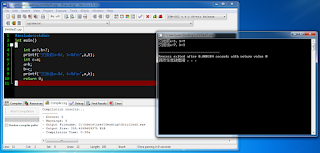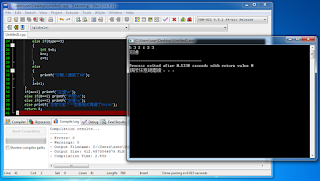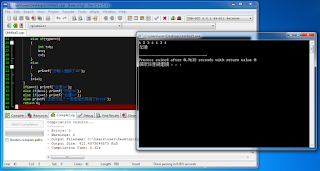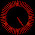## 2016年6月1日 星期三

### 給新手的C++教學 (上冊) - 12. 指標 (Pointer)

#include<cstdio>
int main()
{
int a=3,b=7;
printf("交換前a=%d, b=%d\n",a,b);
int t=a;
a=b;
b=t;
printf("交換後a=%d, b=%d\n",a,b);
return 0;
}我們宣告的兩個變數a、b，它們的資訊被交換了！ (為了方便理解，建議將變數「c」改名為「t」)

↓猜球遊戲↓ (警告：影片有聲音)

$1$代表交換左邊和中間的杯子
$2$代表交換左邊和右邊的杯子
$3$代表交換中間和右邊的杯子

#include<cstdio>
int main()
{
int n;
scanf("%d",&n);
int a=0,b=1,c=0;
int i=0;
while(i<n)
{
int type;
scanf("%d",&type);
if(type==1)
{
int t=a;
a=b;
b=t;
}
else if(type==2)
{
int t=a;
a=c;
c=t;
}
else if(type==3)
{
int t=b;
b=c;
c=t;
}
else
{
printf("你輸入錯誤了XD");
}
i=i+1;
}
if(a==1) printf("左邊\n");
else if(b==1) printf("中間\n");
else if(c==1) printf("右邊\n");
else printf("怎麼可能？一定是程式寫錯了Orz\n");
return 0;
}對於輸入「5 3 2 1 2 3」，程式正確的輸出「右邊」對於輸入「6 2 3 1 1 3 1」，程式正確的輸出「左邊」對於輸入「7 1 1 1 3 2 2 1」，程式正確的輸出「中間」

#include<cstdio>
void Exchange(int x,int y)
{
int t=x;
x=y;
y=t;
}
int main()
{
int n;
scanf("%d",&n);
int a=0,b=1,c=0;
int i=0;
while(i<n)
{
int type;
scanf("%d",&type);
if(type==1)
{
Exchange(a,b);
}
else if(type==2)
{
Exchange(a,c);
}
else if(type==3)
{
Exchange(b,c);
}
else
{
printf("你輸入錯誤了XD");
}
i=i+1;
}
if(a==1) printf("左邊\n");
else if(b==1) printf("中間\n");
else if(c==1) printf("右邊\n");
else printf("怎麼可能？一定是程式寫錯了Orz\n");
return 0;
}對於輸入「5 3 2 1 2 3」，程式錯誤的輸出「中間」對於輸入「6 2 3 1 1 3 1」，程式錯誤的輸出「中間」對於輸入「7 1 1 1 3 2 2 1」，程式正確的輸出「中間」

int x=a,y=b;
int t=x;
x=y;
y=t;

(這時候電腦不會把$a$和$b$的記憶體當作「使用完畢」，因為「Exchange(a,b);」這一行程式碼還是「執行中」的狀態，而且位於$a$和$b$的「作用範圍」裡面)

int a;
int* b=&a;

int a,b,c;
int *d=&a,*e=&b,*f=&c;

void Exchange(int *x,int *y)
{
想辦法交換「x」和「y」所代表的兩塊記憶體的資訊
}

Exchange(&a,&b);

「*」是不是很像射飛鏢在用的「箭靶」呢？這是「箭靶」，有三支「飛鏢」射中箭靶的中心 圖片來源：http://img3.redocn.com/20130429/Redocn_2013042413460991.jpg

「指標」就像一支「飛鏢」一樣
「指標」儲存的資訊 (記憶體編號) 就是這支「飛鏢」指向哪裡

#include<cstdio>
int main()
{
int a=2;
printf("修改前a=%d\n",a);
int *b=&a;
*b=4;
printf("修改後a=%d\n",a);
return 0;
}我們透過「a」的指標「b」，成功修改「a」的數值了！

void Exchange(int *x,int *y)
{
int t=*x;
*x=*y;
*y=t;
}

#include<cstdio>
void Exchange(int *x,int *y)
{
int t=*x;
*x=*y;
*y=t;
}
int main()
{
int n;
scanf("%d",&n);
int a=0,b=1,c=0;
int i=0;
while(i<n)
{
int type;
scanf("%d",&type);
if(type==1)
{
Exchange(&a,&b);
}
else if(type==2)
{
Exchange(&a,&c);
}
else if(type==3)
{
Exchange(&b,&c);
}
else
{
printf("你輸入錯誤了XD");
}
i=i+1;
}
if(a==1) printf("左邊\n");
else if(b==1) printf("中間\n");
else if(c==1) printf("右邊\n");
else printf("怎麼可能？一定是程式寫錯了Orz\n");
return 0;
}對於輸入「5 3 2 1 2 3」，程式正確的輸出「右邊」對於輸入「6 2 3 1 1 3 1」，程式正確的輸出「左邊」對於輸入「7 1 1 1 3 2 2 1」，程式正確的輸出「中間」

#include<cstdio>
int main()
{
int *a=(int*)32405;
*a=911;
return 0;
}

(版權所有 All copyright reserved)

#### 25 則留言:

1.為什麼阿OAOAO

1.咦，請問您在哪裡感到困惑呢？

2.懂了XD

3.那就好 ^_^
有問題隨時歡迎再提出哦～

2.所以如果真的當機重開後就會好嗎？

1.應該會，正常來講XD

3.是不是有一種比較簡單的方式是傳參考呢？

1.2.是的沒錯，只是指標可以做到傳參考做不到的事哦～

3.像是哪些事是傳參考做不到的呢？

4.想請問一下
# define A 3; 跟
const int A = 3;
有甚麼差別嗎？
會建議使用哪一個呢？

1.是有差的
define會把所有符合的字串置換掉，例如#define push_back PB之後s.push_back(v)就可以寫成s.PB(v)
而const int只是宣告一個不能修改的變數而已
因為define的影響範圍廣傷維護性，小莫自己的原則是能用const就用const

5.你好呀，本人嘗試了以下編碼，想大大給點意見 :
#include
int main()
{
while(2>1)
{
int n;
scanf("%d",&n);
if(n==1)
{
printf("Left\n");
}
else if(n==2)
{
printf("Center\n");
}
else if(n==3)
{
printf("Right\n");
}
else if(n>3)
{
printf("Wrong input\n");
}
}
}

1.請問您想要甚麼樣子的建議呢？小莫真的不知道耶XD
然後code的部分可以參考這篇來修正排版哦～

6.現在系統不是都有虛擬記憶體嗎？
電腦會當機嗎？！

1.會不會因為指標存取錯誤導致電腦當機應該跟有沒有虛擬記憶體無關吧？
不過在Windows底下好像記憶體會用權限保護的樣子，如果程式沒有用系統管理員權限執行的話通常不會造成這種悲劇

7.想問傳參考是不是c++獨有的呢？
c是不是沒有？
建議使用傳參考還是傳指標呢？

1.2.是的，傳參考是C++獨有的哦～
建議能用參考就用參考
但是，有些事情是參考做不到的，所以指標也很重要哦～^_^

3.可是這篇好像都只有教傳指標(？

4.哈哈，原本的確是有計畫要撰寫關於「參考(參照)」的教學哦～
可以在這裡找找看(?

8.9.這是C吧？

1.C和C++的確是很像的語言～
但請仔細看，它是C++哦XD

10.真的超級感謝你><
我終於看懂指標是甚麼了